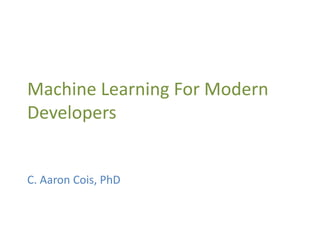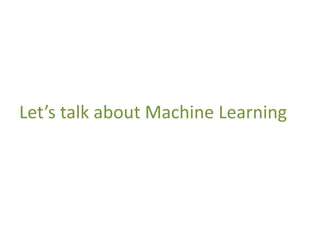Successfully reported this slideshow.

# Machine Learning for Modern Developers×

1 of 66
1 of 66

# Machine Learning for Modern Developers

Slides from my Pittsburgh TechFest 2014 talk, "Machine Learning for Modern Developers". This talk covers basic concepts and math for statistical machine learning, focusing on the problem of classification.

Want some working code from the demos? Head over here: https://github.com/cacois/ml-classification-examples

Slides from my Pittsburgh TechFest 2014 talk, "Machine Learning for Modern Developers". This talk covers basic concepts and math for statistical machine learning, focusing on the problem of classification.

Want some working code from the demos? Head over here: https://github.com/cacois/ml-classification-examples

### Machine Learning for Modern Developers

1. 1. Machine Learning For Modern Developers C. Aaron Cois, PhD
2. 2. Wanna chat? @aaroncois www.codehenge.net github.com/cacois
3. 3. Let’s talk about Machine Learning
4. 4. The Expectation
5. 5. The Sales Pitch
6. 6. The Reaction
7. 7. My Customers
8. 8. The Definition “Field of study that gives computers the ability to learn without being explicitly programmed” ~ Arthur Samuel, 1959
9. 9. That sounds like Artificial Intelligence
10. 10. That sounds like Artificial Intelligence True
11. 11. That sounds like Artificial Intelligence Machine Learning is a branch of Artificial Intelligence
12. 12. That sounds like Artificial Intelligence ML focuses on systems that learn from data Many AI systems are simply programmed to do one task really well, such as playing Checkers. This is a solved problem, no learning required.
13. 13. Isn’t that how Skynet starts?
14. 14. Isn’t that how Skynet starts? Ya, probably
15. 15. Isn’t that how Skynet starts?
16. 16. But it’s also how we do this…
17. 17. …and this…
18. 18. …and this
19. 19. Isn’t this just statistics? Machine Learning can take statistical analyses and make them automated and adaptive Statistical and numerical methods are Machine Learning’s hammer
20. 20. Supervised vs. Unsupervised Supervised = System trained on human labeled data (desired output known) Unsupervised = System operates on unlabeled data (desired output unknown)
21. 21. Supervised learning is all about generalizing a function or mapping between inputs and outputs
22. 22. Supervised Learning Example: Complementary Colors … Training Data … Test Data
23. 23. Supervised Learning Example: Complementary Colors … Training Data f( ) = … Test Data
24. 24. Supervised Learning Example: Complementary Colors … Training Data f( ) = f( ) = … Test Data
25. 25. Let’s Talk Data
26. 26. Supervised Learning Example: Complementary Colors input,output red,green violet,yellow blue,orange orange,blue … training_data.csv red green yellow orange blue … test_data.csv First line indicates data fields
27. 27. Feature Vectors A data point is represented by a feature vector Ninja Turtle = [name, weapon, mask_color] data point 1 = [michelangelo,nunchaku,orange] data point 2 = [leonardo,katana,blue] …
28. 28. Feature Space Feature vectors define a point in an n- dimensional feature space 0 0.1 0.2 0.3 0.4 0.5 0.6 0 0.2 0.4 0.6 0.8 1 1.2 If my feature vectors contain only 2 values, this defines a point in 2-D space: (x,y) = (1.0,0.5)
29. 29. High-Dimensional Feature Spaces Most feature vectors are much higher dimensionality, such as: FVlaptop = [name,screen size,weight,battery life, proc,proc speed,ram,price,hard drive,OS] This means we can’t easily display it visually, but statistics and matrix math work just fine
30. 30. Feature Space Manipulation Feature spaces are important! Many machine learning tasks are solved by selecting the appropriate features to define a useful feature space
31. 31. Task: Classification Classification is the act of placing a new data point within a defined category Supervised learning task Ex. 1: Predicting customer gender through shopping data Ex. 2: From features, classifying an image as a car or truck
32. 32. Linear Classification Linear classification uses a linear combination of features to classify objects
33. 33. Linear Classification Linear classification uses a linear combination of features to classify objects result Weight vector Feature vector Dot product
34. 34. Linear Classification Another way to think of this is that we want to draw a line (or hyperplane) that separates datapoints from different classes
35. 35. Sometimes this is easy Classes are well separated in this feature space Both H1 and H2 accurately separate the classes.
36. 36. Other times, less so This decision boundary works for most data points, but we can see some incorrect classifications
37. 37. Example: Iris Data There’s a famous dataset published by R.A. Fisher in 1936 containing measurements of three types of Iris plants You can download it yourself here: http://archive.ics.uci.edu/ml/datasets/Iris
38. 38. Example: Iris Data Features: 1. sepal length in cm 2. sepal width in cm 3. petal length in cm 4. petal width in cm 5. class Data: 5.1,3.5,1.4,0.2,Iris-setosa 4.9,3.0,1.4,0.2,Iris-setosa … 7.0,3.2,4.7,1.4,Iris-versicolor … 6.8,3.0,5.5,2.1,Iris-virginica …
39. 39. Data Analysis We have 4 features in our vector (the 5th is the classification answer) Which of the 4 features are useful for predicting class?
40. 40. 0 0.5 1 1.5 2 2.5 3 3.5 4 4.5 5 0 1 2 3 4 5 6 7 8 9 sepiawidth sepia length sepia length vs width
41. 41. Different feature spaces give different insight
42. 42. 0 1 2 3 4 5 6 7 8 0 1 2 3 4 5 6 7 8 9 petallength sepia length sepia length vs petal length
43. 43. 0 0.5 1 1.5 2 2.5 3 0 1 2 3 4 5 6 7 8 petalwidth petal length petal length vs petal width
44. 44. 0 0.5 1 1.5 2 2.5 3 0 0.5 1 1.5 2 2.5 3 3.5 4 4.5 5 petalwidth sepia width sepia width vs petal width
45. 45. Half the battle is choosing the features that best represent the discrimination you want
46. 46. Feature Space Transforms The goal is to map data into an effective feature space
47. 47. Demo
48. 48. Logistic Regression Classification technique based on fitting a logistic curve to your data
49. 49. Logistic Regression P(Y | b, x) = 1 1+e-(b0+b1x)
50. 50. Logistic Regression Class 2 Class 1 Probability of data point being in a class Model weights P(Y | b, x) = 1 1+e-(b0+b1x)
51. 51. More Dimensions! Extending the logistic function into N- dimensions:
52. 52. More Dimensions! Extending the logistic function into N- dimensions: Vectors! More weights!
53. 53. Tools Torch7
54. 54. Demo: Logistic Regression (Scikit- Learn) from sklearn.datasets import load_iris from sklearn.linear_model import LogisticRegression iris = load_iris() # set data X, y = iris.data, iris.target # train classifier clf = LogisticRegression().fit(X, y) # 'setosa' data point observed_data_point = [[ 5.0, 3.6, 1.3, 0.25]] # classify clf.predict(observed_data_point) # determine classification probabilities clf.predict_proba(observed_data_point)
55. 55. Learning In all cases so far, “learning” is just a matter of finding the best values for your weights Simply, find the function that fits the training data the best More dimensions more features we can consider
56. 56. What are we doing? Logistic regression is actually maximizing the likelihood of the training data This is an indirect method, but often has good results What we really want is to maximize the accuracy of our model
57. 57. Support Vector Machines (SVMs) Remember how a large number of lines could separate my classes?
58. 58. Support Vector Machines (SVMs) SVMs try to find the optimal classification boundary by maximizing the margin between classes
59. 59. Bigger margins mean better classification of new data points
60. 60. Points on the edge of a class are called Support Vectors Support vectors
61. 61. Demo: Support Vector Machines (Scikit-Learn) from sklearn.datasets import load_iris from sklearn.svm import LinearSVC iris = load_iris() # set data X, y = iris.data, iris.target # run regression clf = LinearSVC().fit(X, y) # 'setosa' data point observed_data_point = [[ 5.0, 3.6, 1.3, 0.25]] # classify clf.predict(observed_data_point)
62. 62. Want to try it yourself? Working code from this talk: https://github.com/cacois/ml- classification-examples
63. 63. Some great online courses Coursera (Free!) https://www.coursera.org/course/ml Caltech (Free!) http://work.caltech.edu/telecourse Udacity (free trial) https://www.udacity.com/course/ud675
64. 64. AMA @aaroncois www.codehenge.net github.com/cacois

### Editor's Notes

• What some customers think
• What some people think
• And like any toolbox, the contents are tools – not processes, procedures, or algorithms. Machine Learning provides these components.
• Supervised learning algorithms are trained on labelled examples, i.e., input where the desired output is known. The supervised learning algorithm attempts to generalise a function or mapping from inputs to outputs which can then be used speculatively to generate an output for previously unseen inputs.

Unsupervised learning algorithms operate on unlabelled examples, i.e., input where the desired output is unknown. Here the objective is to discover structure in the data (e.g. through a cluster analysis), not to generalise a mapping from inputs to outputs.
• Note: many possible boundaries between black and white dots
• plot_iris.py
• DEMO
• i.e. many logistic models can work the same on training data, some are better than others. We can’t tell.